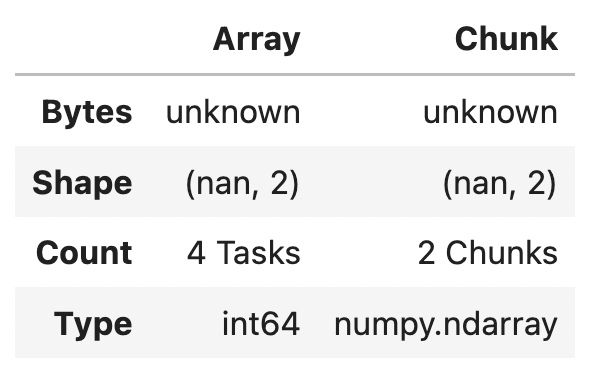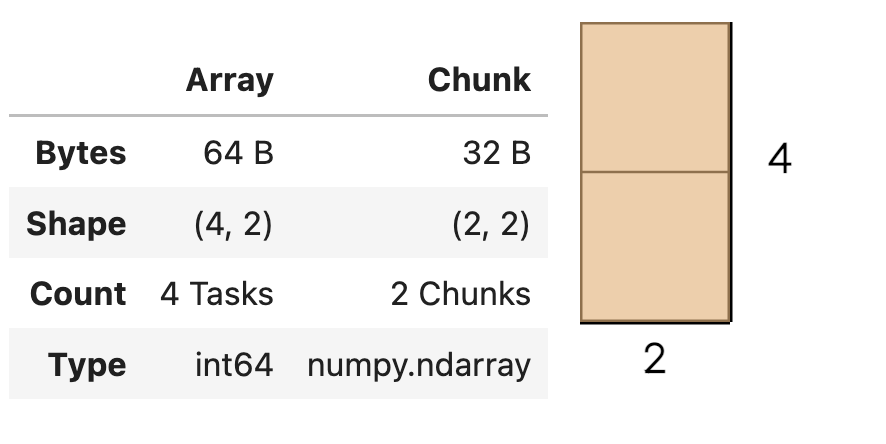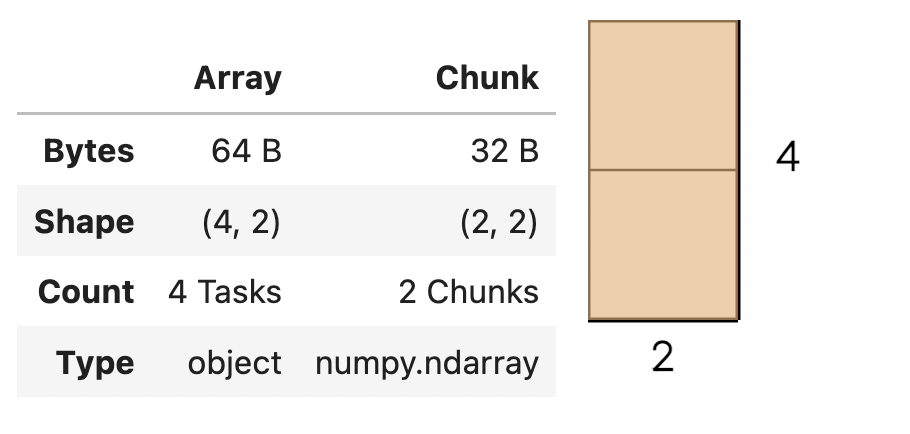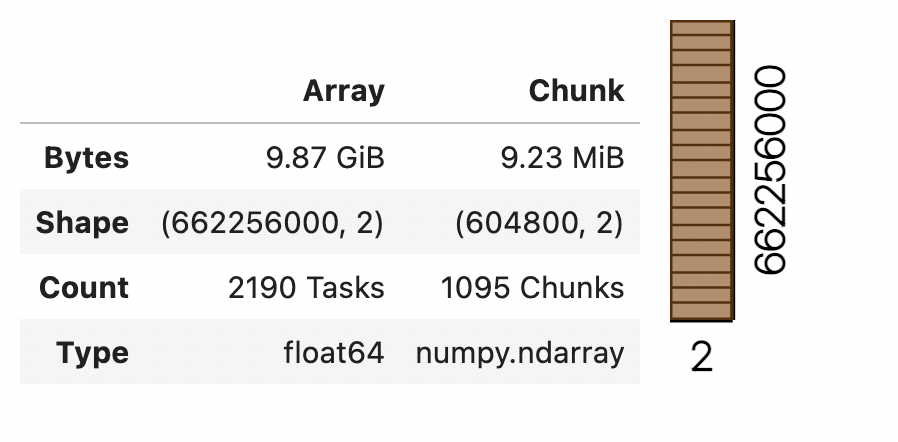June 6, 2022

This post explains how to convert a Dask DataFrame to a Dask Array.  This isn’t an operation you should normally perform, so this post will also explain the limitations and alternate workflows.

Here’s how to create a Dask DataFrame with two partitions that can be converted to a Dask Array:

```import pandas as pd

pdf = pd.DataFrame(
{"num1": [1, 2, 3, 4], "num2": [7, 8, 9, 10]},
)

ddf = dd.from_pandas(pdf, npartitions=2)```

```>>> my_arr = ddf.to_dask_array()
>>> my_arr```You can run `compute` to see the values that are contained in the array:

```>>> my_arr.compute()

array([[ 1,  7],
[ 2,  8],
[ 3,  9],
[ 4, 10]])```

This isn’t ideal because Dask doesn’t know the shape of the chunks.  This can cause later computations to error out.  Let’s see how to force Dask to compute the shape of the chunks when converting from a DataFrame to an array.

You can set the `length` property when converting from a Dask DataFrame to a Dask Array to have Dask immediately compute the length of each partition, so it knows the shape of each chunk.

```>>> my_arr = ddf.to_dask_array(lengths=True)
>>> my_arr```You can also manually specify a list of chunk sizes, but these aren’t verified, so they may cause downstream computations to error out.

Let’s create a Dask DataFrame with columns that have different data types and see what happens when we try to convert it to a Dask Array.  Start by creating the Dask DataFrame.

```pdf = pd.DataFrame(
{"num": [1, 2, 3, 4], "letter": ["a", "b", "c", "d"]},
)

ddf = dd.from_pandas(pdf, npartitions=2)```

```>>> my_arr = ddf.to_dask_array(lengths=True)
>>> my_arr```The Dask Array has the `object` type.  Heterogeneous data must be stored as objects in Dask Array.

You can also see this data type when running `compute:`

```>>> my_arr.compute()

array([[1, 'a'],
[2, 'b'],
[3, 'c'],
[4, 'd']], dtype=object)```

Create a Dask cluster so we can read a large dataset into a Dask DataFrame and then convert it to a Dask Array.

```import coiled

cluster = coiled.Cluster(name="powers-crt-003", software="crt-003", n_workers=5)

Read in a Parquet dataset into a Dask DataFrame and inspect the column data types.

```>>> ddf = dd.read_parquet(
"s3://coiled-datasets/timeseries/20-years/parquet",
storage_options={"anon": True, "use_ssl": True},
)

>>> ddf.dtypes

id        int64
name     object
x       float64
y       float64
dtype: object```

The `x` and `y` columns are both `float64`.  Let’s convert those two columns to a Dask Array.

```>>> ddf = dd.read_parquet(
"s3://coiled-datasets/timeseries/20-years/parquet",
storage_options={"anon": True, "use_ssl": True},
columns=["x", "y"],
)

>>> some_arr```The Dask Array has 662 million rows, but each chunk only has 604,800 rows.  The data is nicely spread out across multiple chunks.

The example we just saw is a good example of when converting a Dask DataFrame to a Dask Array may be justified.  You cannot read Parquet files directly into Dask Arrays.  Reading them into a Dask DataFrame first and then converting them to a Dask Array may be your best option.

If you have to perform this analysis in production it’s probably best to first convert the data to a Dask Array compliant file format before performing the actual array analysis.  Here’s the full workflow: